Скачать презентацию experiment 8 minor Losses exp 8

756eef67c37c8b8e1b548cd7fbc050f6.ppt

• Количество слайдов: 26experiment 8 : minor Losses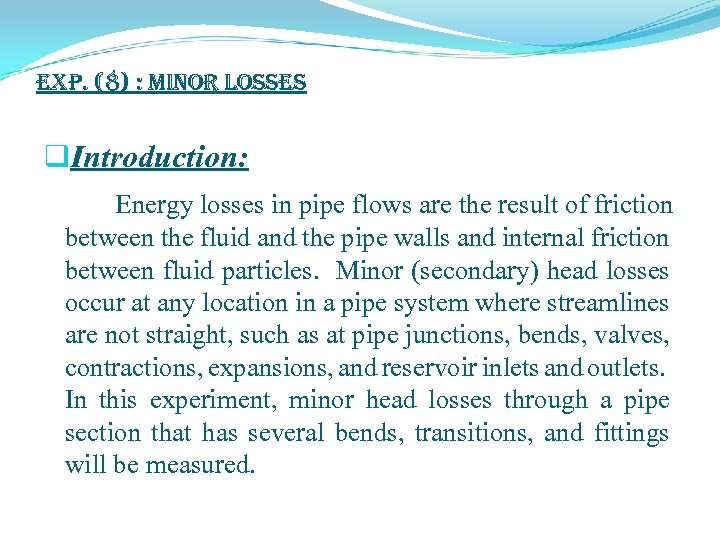exp. (8) : Minor Losses q. Introduction: Energy losses in pipe flows are the result of friction between the fluid and the pipe walls and internal friction between fluid particles. Minor (secondary) head losses occur at any location in a pipe system where streamlines are not straight, such as at pipe junctions, bends, valves, contractions, expansions, and reservoir inlets and outlets. In this experiment, minor head losses through a pipe section that has several bends, transitions, and fittings will be measured.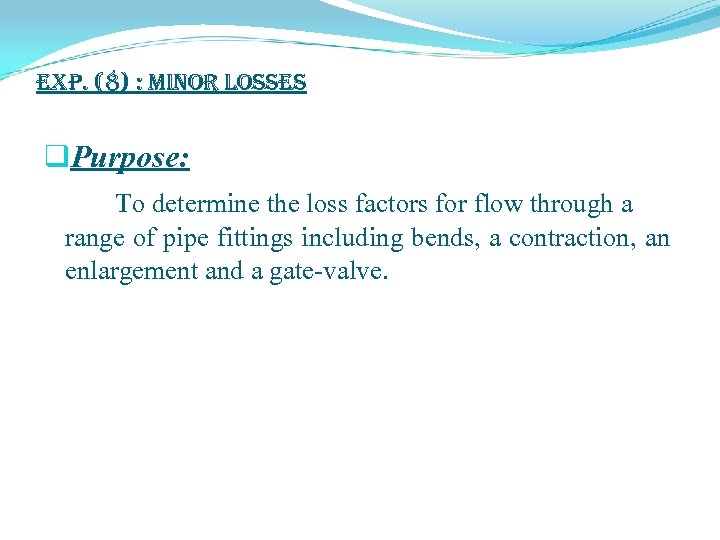exp. (8) : Minor Losses q. Purpose: To determine the loss factors for flow through a range of pipe fittings including bends, a contraction, an enlargement and a gate-valve.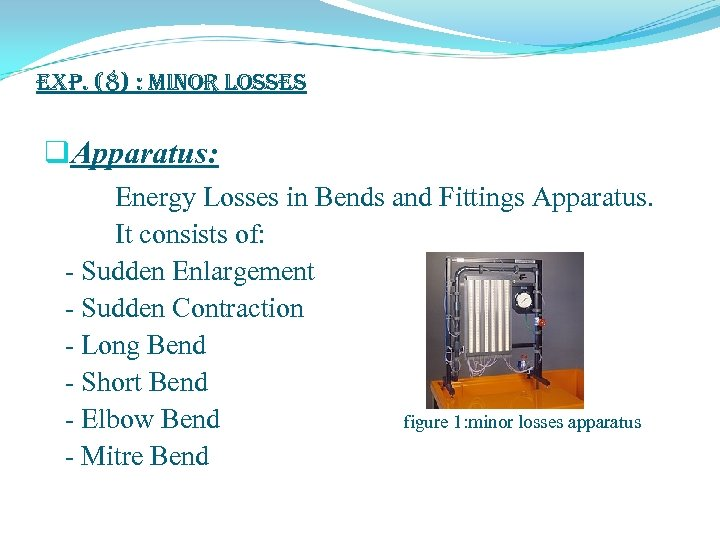exp. (8) : Minor Losses q. Apparatus: Energy Losses in Bends and Fittings Apparatus. It consists of: - Sudden Enlargement - Sudden Contraction - Long Bend - Short Bend - Elbow Bend figure 1: minor losses apparatus - Mitre Bend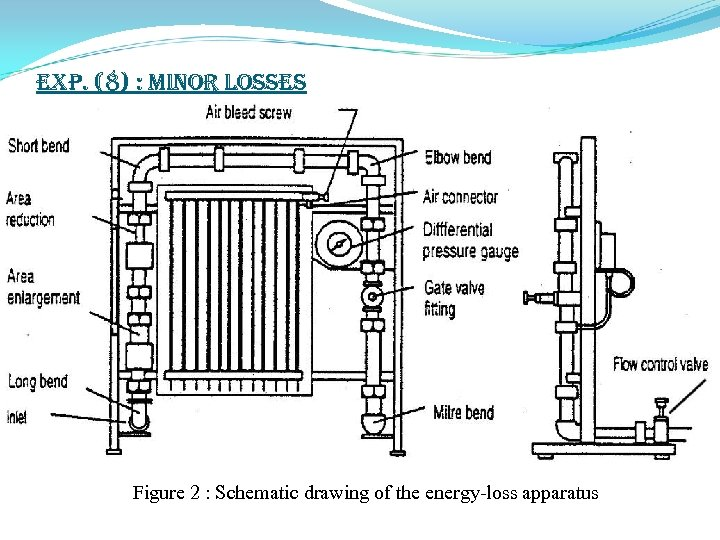exp. (8) : Minor Losses Figure 2 : Schematic drawing of the energy-loss apparatus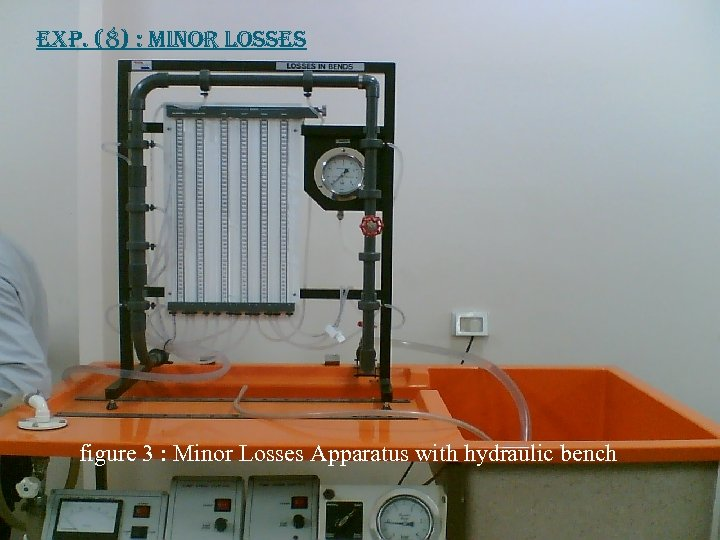exp. (8) : Minor Losses figure 3 : Minor Losses Apparatus with hydraulic bench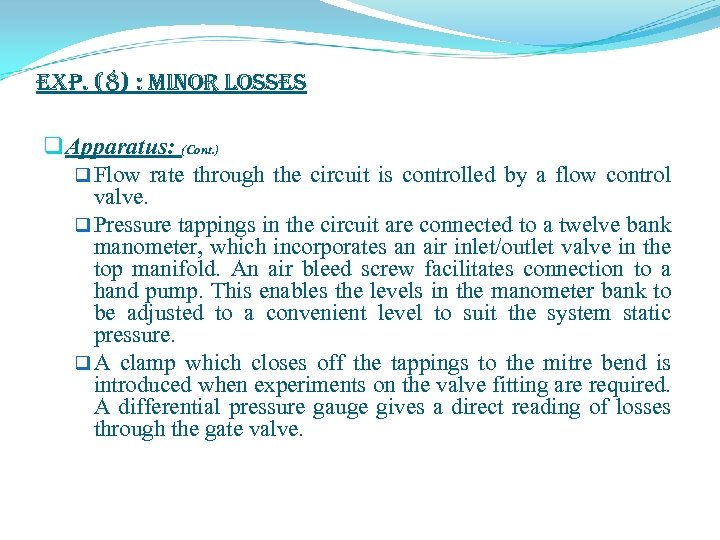exp. (8) : Minor Losses q Apparatus: (Cont. ) q Flow rate through the circuit is controlled by a flow control valve. q Pressure tappings in the circuit are connected to a twelve bank manometer, which incorporates an air inlet/outlet valve in the top manifold. An air bleed screw facilitates connection to a hand pump. This enables the levels in the manometer bank to be adjusted to a convenient level to suit the system static pressure. q A clamp which closes off the tappings to the mitre bend is introduced when experiments on the valve fitting are required. A differential pressure gauge gives a direct reading of losses through the gate valve.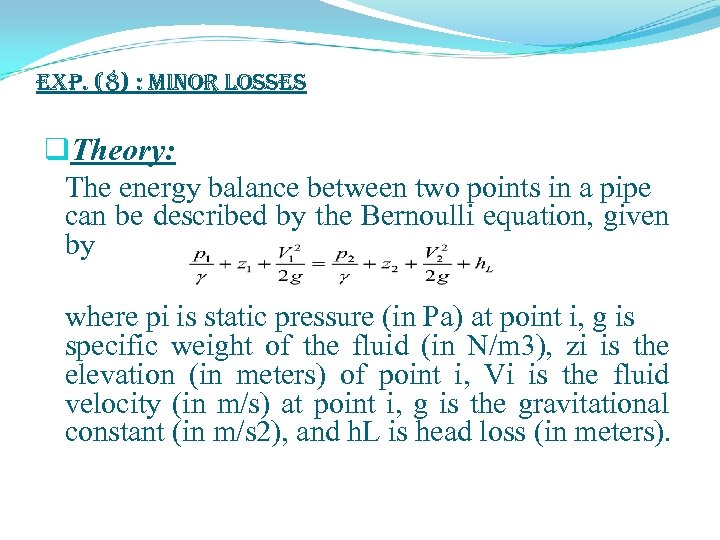exp. (8) : Minor Losses q. Theory: The energy balance between two points in a pipe can be described by the Bernoulli equation, given by where pi is static pressure (in Pa) at point i, g is specific weight of the fluid (in N/m 3), zi is the elevation (in meters) of point i, Vi is the fluid velocity (in m/s) at point i, g is the gravitational constant (in m/s 2), and h. L is head loss (in meters).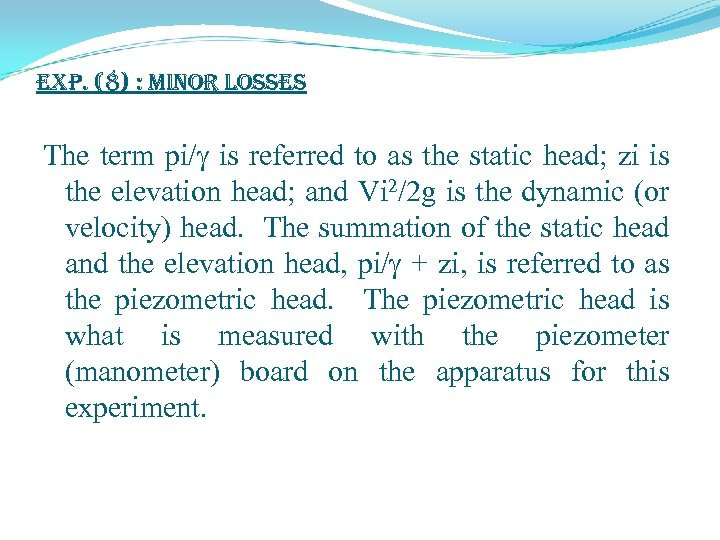exp. (8) : Minor Losses The term pi/ is referred to as the static head; zi is the elevation head; and Vi 2/2 g is the dynamic (or velocity) head. The summation of the static head and the elevation head, pi/ + zi, is referred to as the piezometric head. The piezometric head is what is measured with the piezometer (manometer) board on the apparatus for this experiment.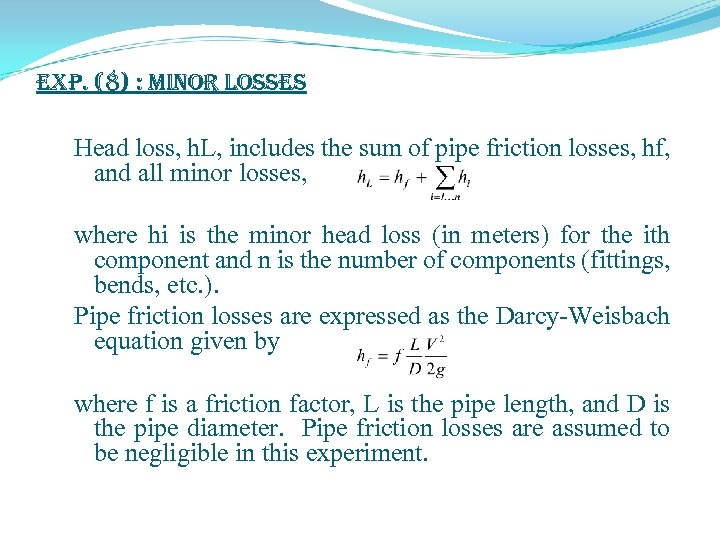exp. (8) : Minor Losses Head loss, h. L, includes the sum of pipe friction losses, hf, and all minor losses, where hi is the minor head loss (in meters) for the ith component and n is the number of components (fittings, bends, etc. ). Pipe friction losses are expressed as the Darcy-Weisbach equation given by where f is a friction factor, L is the pipe length, and D is the pipe diameter. Pipe friction losses are assumed to be negligible in this experiment.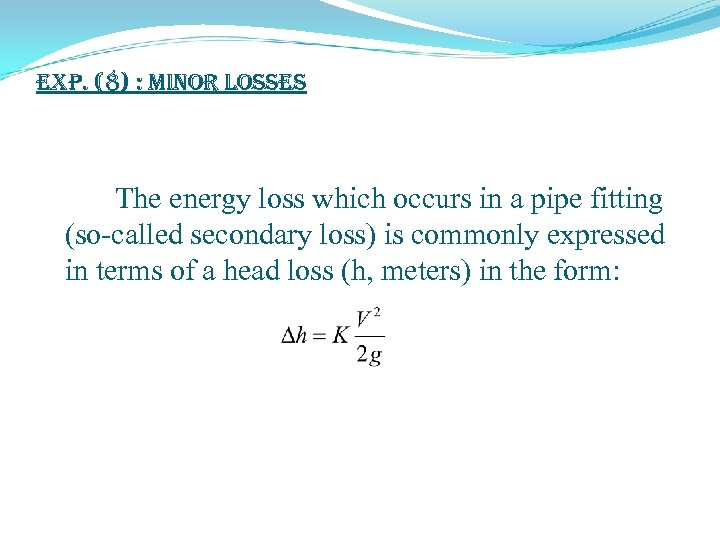exp. (8) : Minor Losses The energy loss which occurs in a pipe fitting (so-called secondary loss) is commonly expressed in terms of a head loss (h, meters) in the form: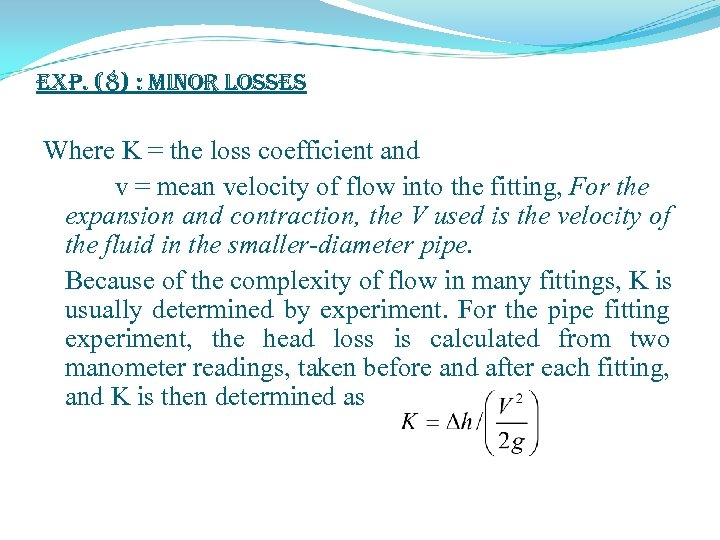exp. (8) : Minor Losses Where K = the loss coefficient and v = mean velocity of flow into the fitting, For the expansion and contraction, the V used is the velocity of the fluid in the smaller-diameter pipe. Because of the complexity of flow in many fittings, K is usually determined by experiment. For the pipe fitting experiment, the head loss is calculated from two manometer readings, taken before and after each fitting, and K is then determined as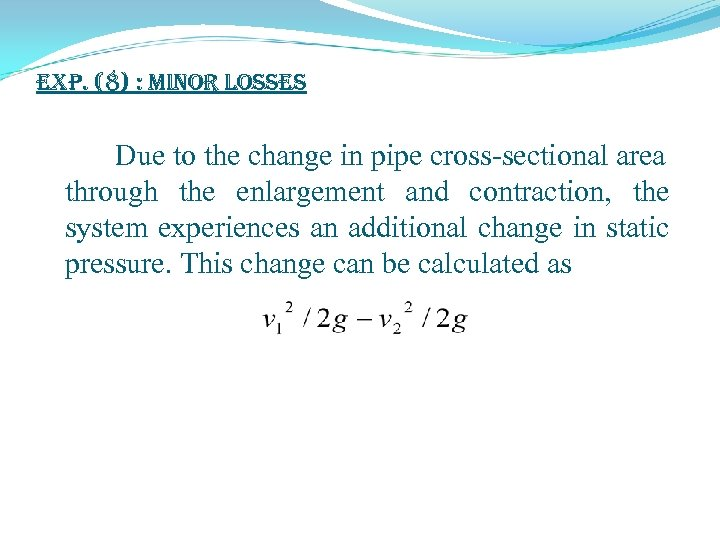exp. (8) : Minor Losses Due to the change in pipe cross-sectional area through the enlargement and contraction, the system experiences an additional change in static pressure. This change can be calculated as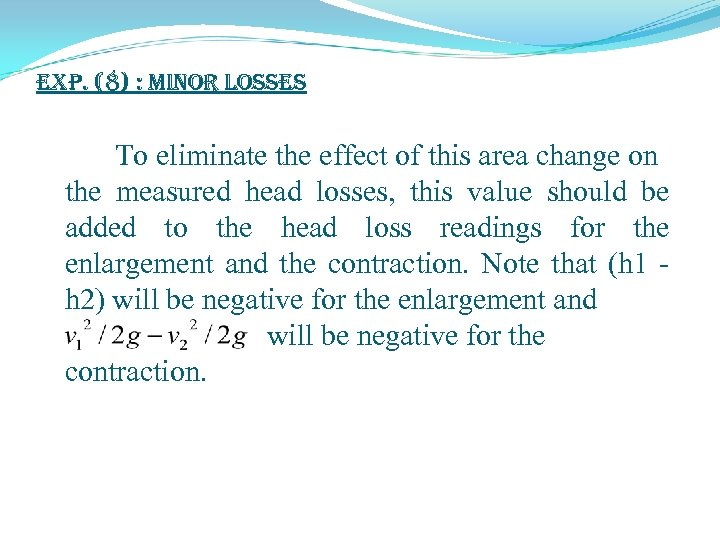exp. (8) : Minor Losses To eliminate the effect of this area change on the measured head losses, this value should be added to the head loss readings for the enlargement and the contraction. Note that (h 1 - h 2) will be negative for the enlargement and will be negative for the contraction.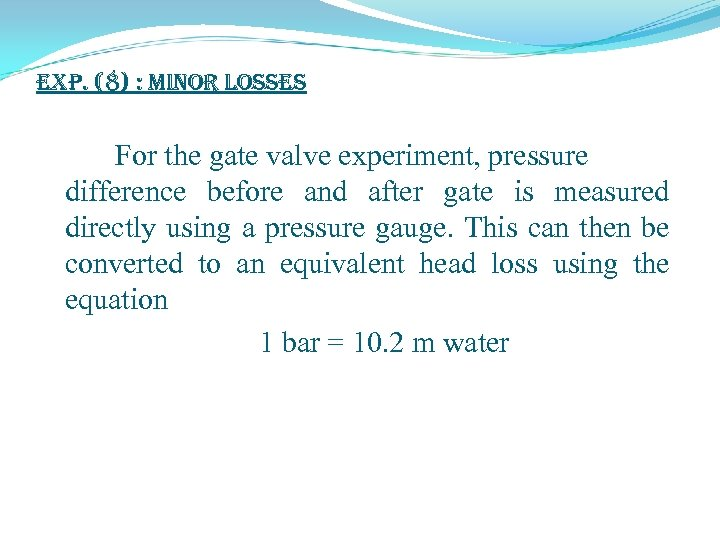exp. (8) : Minor Losses For the gate valve experiment, pressure difference before and after gate is measured directly using a pressure gauge. This can then be converted to an equivalent head loss using the equation 1 bar = 10. 2 m water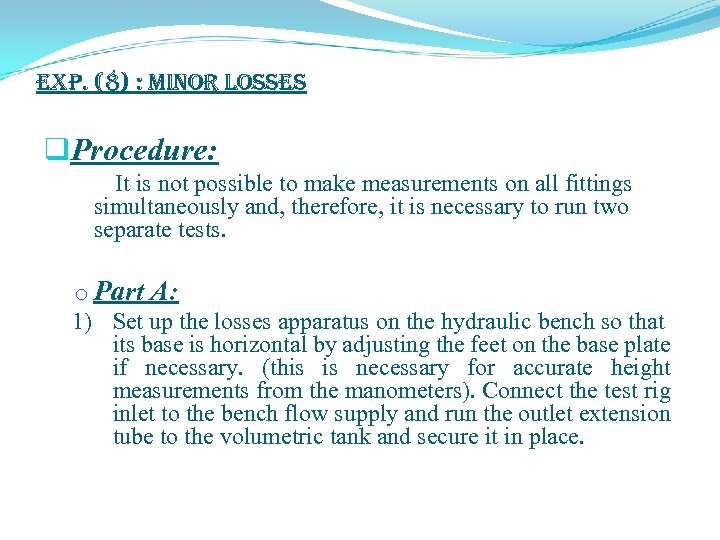exp. (8) : Minor Losses q. Procedure: It is not possible to make measurements on all fittings simultaneously and, therefore, it is necessary to run two separate tests. o Part A: 1) Set up the losses apparatus on the hydraulic bench so that its base is horizontal by adjusting the feet on the base plate if necessary. (this is necessary for accurate height measurements from the manometers). Connect the test rig inlet to the bench flow supply and run the outlet extension tube to the volumetric tank and secure it in place.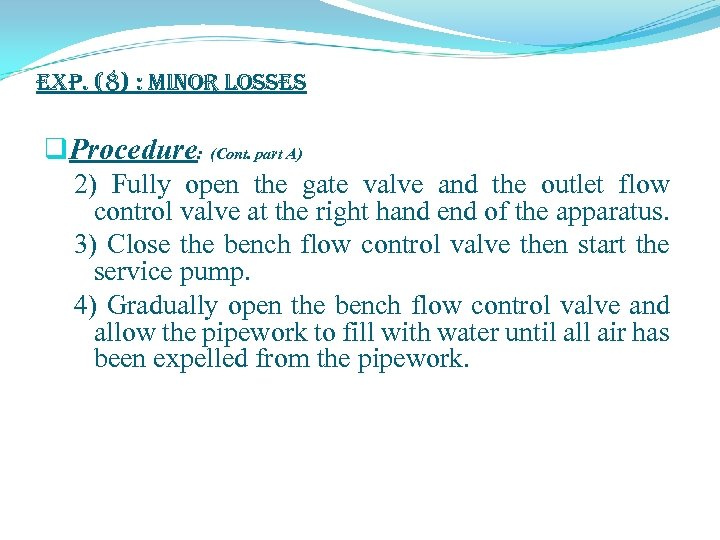exp. (8) : Minor Losses q. Procedure: (Cont. part A) 2) Fully open the gate valve and the outlet flow control valve at the right hand end of the apparatus. 3) Close the bench flow control valve then start the service pump. 4) Gradually open the bench flow control valve and allow the pipework to fill with water until all air has been expelled from the pipework.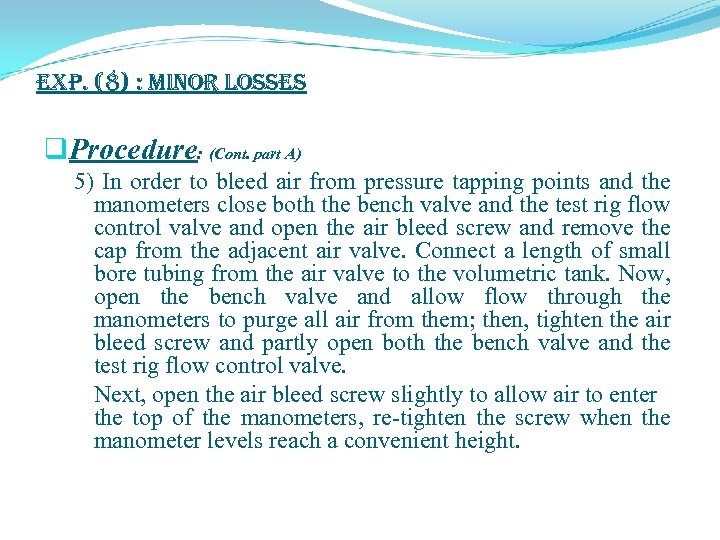exp. (8) : Minor Losses q. Procedure: (Cont. part A) 5) In order to bleed air from pressure tapping points and the manometers close both the bench valve and the test rig flow control valve and open the air bleed screw and remove the cap from the adjacent air valve. Connect a length of small bore tubing from the air valve to the volumetric tank. Now, open the bench valve and allow flow through the manometers to purge all air from them; then, tighten the air bleed screw and partly open both the bench valve and the test rig flow control valve. Next, open the air bleed screw slightly to allow air to enter the top of the manometers, re-tighten the screw when the manometer levels reach a convenient height.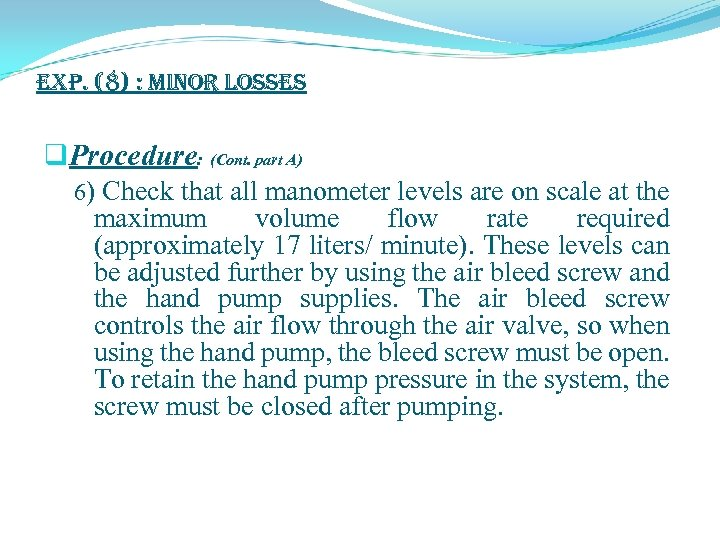exp. (8) : Minor Losses q. Procedure: (Cont. part A) 6) Check that all manometer levels are on scale at the maximum volume flow rate required (approximately 17 liters/ minute). These levels can be adjusted further by using the air bleed screw and the hand pump supplies. The air bleed screw controls the air flow through the air valve, so when using the hand pump, the bleed screw must be open. To retain the hand pump pressure in the system, the screw must be closed after pumping.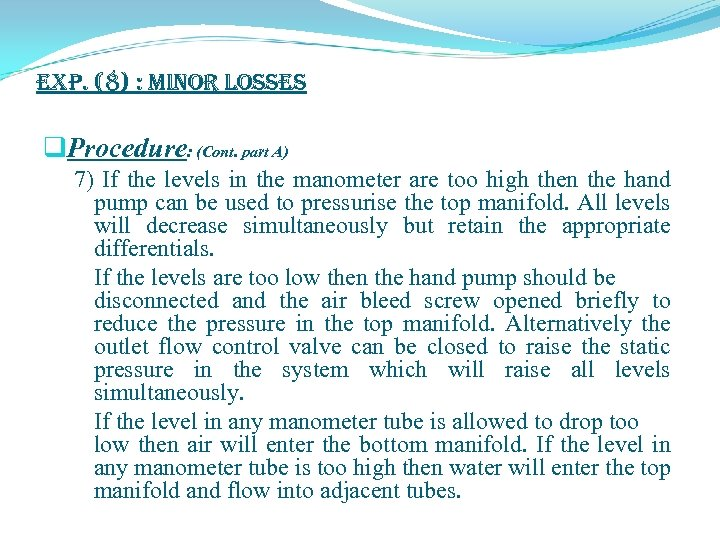exp. (8) : Minor Losses q. Procedure: (Cont. part A) 7) If the levels in the manometer are too high then the hand pump can be used to pressurise the top manifold. All levels will decrease simultaneously but retain the appropriate differentials. If the levels are too low then the hand pump should be disconnected and the air bleed screw opened briefly to reduce the pressure in the top manifold. Alternatively the outlet flow control valve can be closed to raise the static pressure in the system which will raise all levels simultaneously. If the level in any manometer tube is allowed to drop too low then air will enter the bottom manifold. If the level in any manometer tube is too high then water will enter the top manifold and flow into adjacent tubes.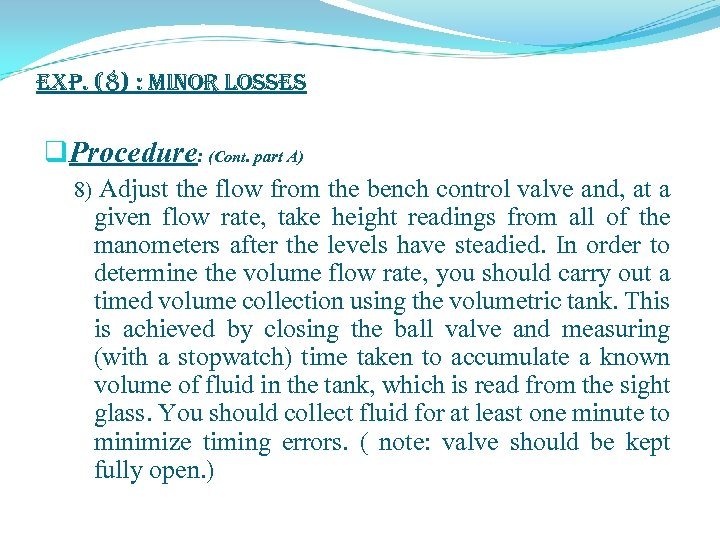exp. (8) : Minor Losses q. Procedure: (Cont. part A) 8) Adjust the flow from the bench control valve and, at a given flow rate, take height readings from all of the manometers after the levels have steadied. In order to determine the volume flow rate, you should carry out a timed volume collection using the volumetric tank. This is achieved by closing the ball valve and measuring (with a stopwatch) time taken to accumulate a known volume of fluid in the tank, which is read from the sight glass. You should collect fluid for at least one minute to minimize timing errors. ( note: valve should be kept fully open. )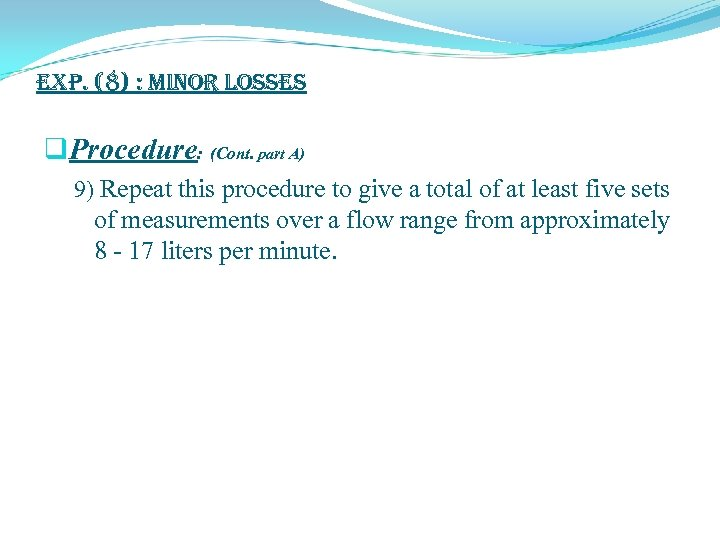exp. (8) : Minor Losses q. Procedure: (Cont. part A) 9) Repeat this procedure to give a total of at least five sets of measurements over a flow range from approximately 8 - 17 liters per minute.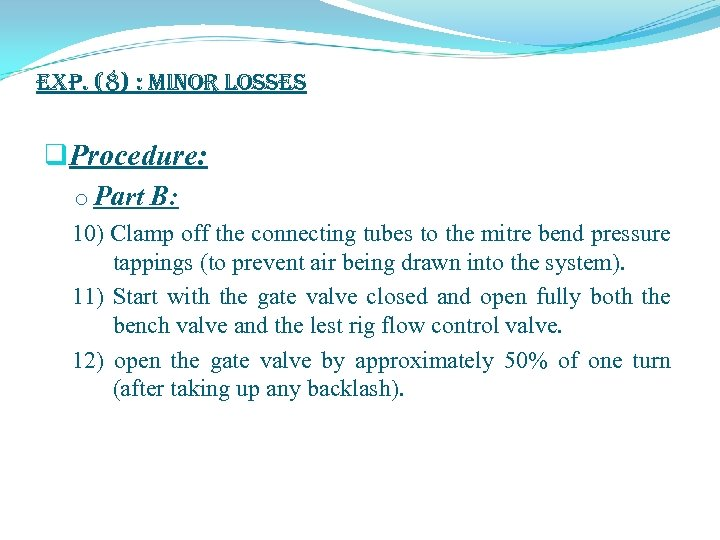exp. (8) : Minor Losses q. Procedure: o Part B: 10) Clamp off the connecting tubes to the mitre bend pressure tappings (to prevent air being drawn into the system). 11) Start with the gate valve closed and open fully both the bench valve and the lest rig flow control valve. 12) open the gate valve by approximately 50% of one turn (after taking up any backlash).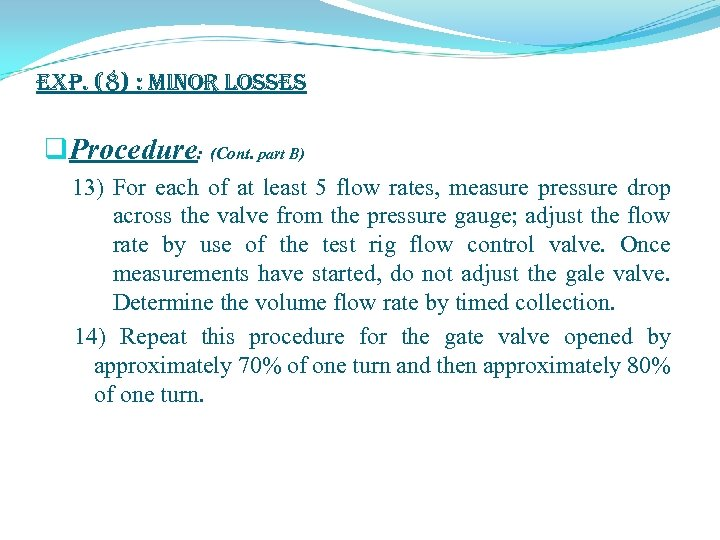exp. (8) : Minor Losses q. Procedure: (Cont. part B) 13) For each of at least 5 flow rates, measure pressure drop across the valve from the pressure gauge; adjust the flow rate by use of the test rig flow control valve. Once measurements have started, do not adjust the gale valve. Determine the volume flow rate by timed collection. 14) Repeat this procedure for the gate valve opened by approximately 70% of one turn and then approximately 80% of one turn.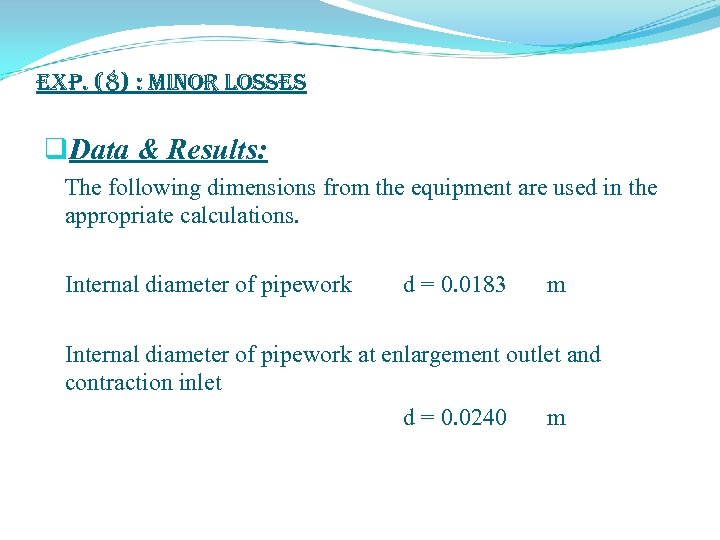exp. (8) : Minor Losses q. Data & Results: The following dimensions from the equipment are used in the appropriate calculations. Internal diameter of pipework d = 0. 0183 m Internal diameter of pipework at enlargement outlet and contraction inlet d = 0. 0240 mexp. (8) : Minor Losses q. Data & Results: Tables. doc Minor Losses (excel)